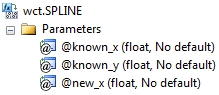# SQL Server cubic spline interpolation

SPLINE

Updated: 30 April 2011

Note: This documentation is for the SQL2008 (and later) version of this XLeratorDB function, it is not compatible with SQL Server 2005.
Click here for the SQL2005 version of the SPLINE function

Use SPLINE to calculate the interpolated value of y given x in the array (x, y). SPLINE uses cubic spline interpolation. Unlike the scalar range query SPLINE function from earlier versions of XLeratorDB/math, the aggregate SPLINE function is not sensitive to the order of the array (x, y).
SyntaxArguments
@known_x
the x-values to be used in the interpolation calculation. @known_x must be of the type float or of a type that implicitly converts to float.
@known_y
the y-values to be used in the interpolation calculation. @known_y must be of the type float or of a type that implicitly converts to float.
@new_x
the new x-value for which you want INTERP to calculate the y-value.
Return Types
float
Remarks
·         For linear interpolation use the INTERP function.
·         For polynomial interpolation use the POLYINTERP function.
·         If an x-y pair contains a NULL, then the pair is not used in the interpolation calculation.
·         SPLINE will accept values for @new_x that are less than the minimum @known_x or greater than the maximum @known_x.
·         @new_x must remain invariant for the GROUP.
Examples
Given the following x- and y-values, we want to calculate the y-value for x = 0.75
SET NOCOUNT ON

SELECT wct.SPLINE(x,y,0.75) as SPLINE
FROM (VALUES
(0.5,0.479426),
(1,0.841471),
(1.5,0.997495),
(2,0.909297),
(2.5,0.598472),
(3,0.14112),
(3.5,-0.350783),
(4,-0.756802)
) n(x,y)

This produces the following result.
SPLINE
----------------------
0.675926077765373

In this example, we will interpolate using dates.
SET NOCOUNT ON

SELECT cast(x as datetime) as x,y
INTO #a
FROM (VALUES
('2012-Apr-30',0.0028),
('2013-Apr-30',0.0056),
('2014-Apr-30',0.0085),
('2016-Apr-30',0.0164),
('2018-Apr-30',0.0235),
('2021-Apr-30',0.0299),
('2031-Apr-30',0.0382),
('2041-Apr-30',0.0406)
) m(x, y)

SELECT wct.SPLINE(cast(x as float), y, cast(Cast('2012-Oct-31' as datetime) as float)) as SPLINE
from #a

DROP TABLE #a

This produces the following result.
SPLINE
----------------------
0.0042210614815398

We could have used the YEARFRAC function from XLeratorDB/financial, which makes the SQL somewhat simpler, but also permits interpolation using different interest bases. In this example, we will use YEARFRAC, using the 3-part function identifier because the YEARFRAC function is contained in a different database, to interpolate using the BOND interest basis.
SET NOCOUNT ON

SELECT cast(x as datetime) as x,y
INTO #a
FROM (VALUES
('2012-Apr-30',0.0028),
('2013-Apr-30',0.0056),
('2014-Apr-30',0.0085),
('2016-Apr-30',0.0164),
('2018-Apr-30',0.0235),
('2021-Apr-30',0.0299),
('2031-Apr-30',0.0382),
('2041-Apr-30',0.0406)
) m(x, y)

SELECT wct.SPLINE(
westclintech.wct.YEARFRAC('2011-Apr-30',x,0)
,y
,westclintech.wct.YEARFRAC('2011-Apr-30','2012-Oct-31',0)
) as SPLINE
FROM #a

DROP TABLE #a
This produces the following result.
SPLINE
----------------------
0.00420966880550746

In this example, we provide a @new_x value that is less than the minimum @known_x value.
SET NOCOUNT ON

SELECT wct.SPLINE(x, y, -3) as SPLINE
FROM (VALUES
(-2,-2),
(-1,-0.5),
(0,1),
(1,2.5),
(2,4),
(3,5.5)
) n(x, y)
This produces the following result.
SPLINE
----------------------
-3.5

In this example, we provide a @new_x value that is greater than the maximum @known_x value.
SET NOCOUNT ON

SELECT wct.SPLINE(x, y, 4) as SPLINE
FROM (VALUES
(-2,-2),
(-1,-0.5),
(0,1),
(1,2.5),
(2,4),
(3,5.5)
) n(x, y)
This produces the following result.
SPLINE
----------------------
7

In this example, one of the @known_y values is NULL.
SET NOCOUNT ON

SELECT wct.SPLINE(x, y, 1.5) as SPLINE
FROM (VALUES
(-2,-2),
(-1,-0.5),
(0,1),
(1,NULL),
(2,4),
(3,5.5)
) n(x, y)
This produces the following result.
SPLINE
----------------------
3.25

In this example, one of the @known_x values is NULL.
SET NOCOUNT ON

SELECT wct.SPLINE(x, y, 1.5) as SPLINE
FROM (VALUES
(-2,-2),
(-1,-0.5),
(0,1),
(1,2.5),
(NULL,4),
(3,5.5)
) n(x, y)
This produces the following result.
SPLINE
----------------------

3.25

### PricingCopyright 2008-2021 Westclintech LLC         Privacy Policy        Terms of Service